##Circumconics of the triangle -II

From the many ways to define a circumconic of a triangle we examine here one, related to the other method discussed in TriangleCircumconics.html . There we used for each circumconic a different projectivity to map the circumcircle onto the conic under discussion. Here we fix a unique projectivity F, used to get all circumconics of the triangle A'B'C'. The projectivity F is uniquely defined by the properties to map (i) the circumcircle of the fixed equilateral ABC to the circumcircle of the triangle A'B'C' and (ii) to map vertices A, B, C to A', B', C'. Then to obtain a circumconic of A'B'C', simply apply F to a circumconic of ABC.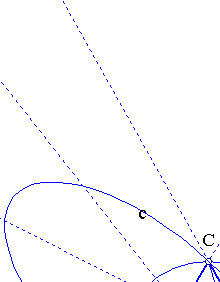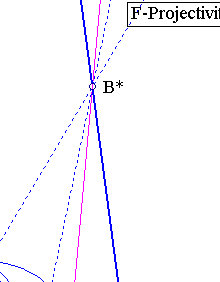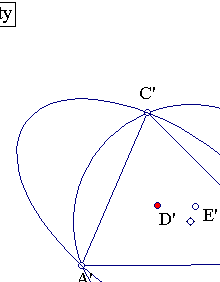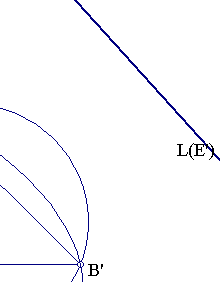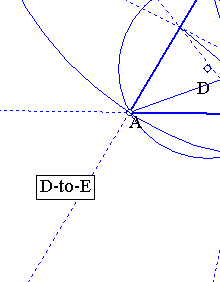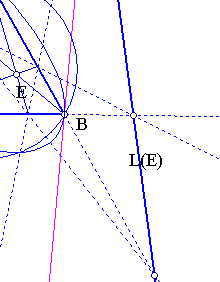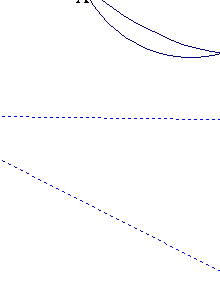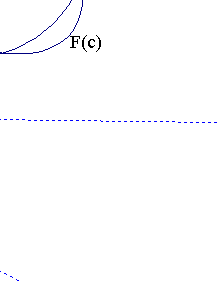Notice that the circumconics of the equilateral can be obtained through the various projectivities (denoted here by D-to-E) discussed in TriangleCircumconics.html . Notice also that the center D of the equilateral maps under F to the symmedian point of A'B'C' and the line at infinity (trilinear polar of D) maps to the Lemoine line of A'B'C' (trilinear polar of D').
Since F is not fixing the line at infinity it is not an affinity. In particular ratios of areas are not preserved and the outer Steiner ellipse of triangle ABC, which is its circumcircle is not mapped to the outer Steiner ellipse of A'B'C'. To obtain this conic one has to take E such that the corresponding trilinear polar L(E) maps under F (i.e. line L(E')) to the line at infinity.
The file ProjectivityFixingVertices.html contains a study of the projectivity D-to-E for the case of the equilateral.